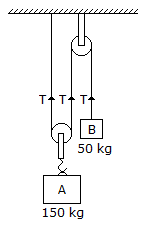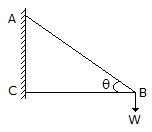Last updated: August 14, 2020

1. The ratio of static friction to dynamic friction is always
2. The unit of force in S.I. system of units is
3. The efficiency of a lifting machine is the ratio of
4. In order to determine the effects of a force, acting on a body, we must know
5. A body is said to move or vibrate with simple harmonic motion if its acceleration is directed towards the mean position.
6. Coefficient of friction depends upon
7. The moment of inertia of a solid cone of mass 'm' and base radius 'r' about its vertical axis is
8. Two blocks A and B of masses 150 kg and 50 kg respectively are connected by means of a string as shown in the below figure. The tension in all the three strings __________ be same.9. The force induced in the string AB due to the load W, as shown in the below figure is10. The kinetic energy of a body __________ upon its mass and velocity.
11. Non-coplaner non-concurrent forces are those forces which
12. A spherical body is symmetrical about its perpendicular axis. According to Routh's rule, the moment of inertia of a body about an axis passing through its centre of gravity is (where, M = Mass of the body, and S = Sum of the squares of the two semi-axes.)
13. A lifting machine lifts a load of 1000N through a distance of 0.2 m by means of an effort of 200N through a distance of 1m. This machine is an ideal one.
14. One kg force is equal to
15. The three forces of 100 N, 200 N and 300 N have their lines of action parallel to each other but act in the opposite directions. These forces are known as
16. A force while acting on a body may
17. The Cartesian equation of trajectory is(where u = Velocity of projection, α = Angle of projection, and x, y = Co-ordinates of any point on the trajectory after t seconds.)
18. The maximum displacement of a body, from its mean position is called amplitude.
19. A pendulum which executes one beat per second is known as
20. A screw jack used for lifting the loads is
Engineering Mechanics MCQ 14
You got {{userScore}} out of {{maxScore}} correct
{{title}}
{{image}}
{{content}}